telescopeѲptics.net          ▪▪▪▪                                             CONTENTS

As telescopes evolved, it has been discovered that performance of all-reflecting systems - especially those with more strongly curved surfaces - can be improved if mirrors are combined with lens elements. General term for telescope systems using both, reflective and refractive elements in order to form the image is catadioptric. Main working principle of a catadioptric telescope is that aberrations of reflecting and refracting elements cancel each other (kata=against, dioptric=refractive). Lens element(s) used in combination with mirrors can be either placed in the path of incoming light, when it is a full-aperture corrector, or in the converging cone produced by mirror element(s), when it is a sub-aperture corrector.

Strictly talking, catadioptric telescope is designed as a synergy of reflective and refractive elements, requiring both for its functioning. Such design is sometimes called true catadioptric. That separates it from hybrid catadioptric, usually a mirror telescope using refractive field-corrector, which can function without its refractive component.

Following this general dividing line, catadioptric telescopes are described within their two main groups, those with sub-aperture and those with full-aperture lens corrector. The former can be either true or hybrid catadioptric systems, while the latter are usually "true catadioptrics", requiring the lens element for functioning.

Aberrations of a full-aperture refractive corrector nearly coinciding with the aperture stop - which is the usual scenario - are those of a lens for object at infinity. Also, aperture stop usually shifted from primary mirror to the full-aperture corrector is a factor that can affect the aberrations of mirror surfaces following it in the optical train.

On the other hand, sub-aperture lens corrector, usually placed in the light converging after reflection(s) from mirror element(s), doesn't affect the aberrations of mirror surface(s). Its object is the image formed by preceding mirror element, and its aperture stop is the exit pupil formed by this element. This makes aberration relations for sub-aperture corrector different - generally more complex - than those for full-aperture corrector. It is illustrated by aberration relations for sub-aperture corrector lens element that follow.

# 10.1.1. Sub-aperture corrector aberrations

Unlike telescope objectives and most full-aperture corrector arrangements, sub-aperture correctors are usually positioned in a converging light cone. In other words, these lens correctors are preceded by one or more optical surfaces. Consequently, their aperture stop is located at the exit pupil formed by the preceding element. In a Newtonian both, entrance and exit pupil coincide at the primary mirror's surface, which is also the stop position for sub-aperture corrector. In a two-mirror system, the stop is at the image of primary formed by the secondary.

Stop shift doesn't affect axial cone width or position at the corrector, thus the expression for spherical aberration remains unchanged. For off-axis cones, stop shift causes the chief ray to shift off the center of corrector's front surface. In other words, converging wavefront passes through different portion of the corrector than in the case when the stop is at the front surface. As a result, expressions for off-axis aberration of sub-aperture corrector are different - generally more complex - than those for the lens objective with the stop at the surface.

This also applies to the secondary mirror in two-mirror systems. The difference is that lens correctors have more surfaces - commonly four or more - each contributing its own aberrations. Consequently, the appropriate aberration calculation is significantly more complex. For that reason, the consideration here will be limited to a general form of aberration relations for a single lens, showing how the three point-image quality aberrations, spherical, coma and astigmatism, as well as field curvature, are affected and inter-related at a sub-aperture lens corrector.

Axial correction

Aberration coefficient for lower-order (primary) spherical aberration of sub-corrector lens element is the same as for a single lens element in general: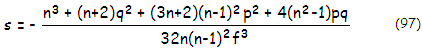with n being the refractive index, q=(R2+R1)/(R2-R1) the lens shape factor, and p=(2ƒ/O)-1=1-2ƒ/I the lens position factor (ƒ is the lens focal length, O the object distance and I the image-lens separation). Obviously, only the value of p is different from those for object at infinity.

The peak aberration coefficient, which with primary spherical aberration equals the P-V wavefront error at paraxial focus, is S=W=sD4/16, D being the aperture diameter (the wavefront error at the best focus is smaller by a factor of 0.25, or W=sD4/64). For the front lens, the object is the image formed by the preceding mirror element (or elements), while the object for the rear lens is image formed by the front lens. The combined error varies with the lens separation, which directly determines relative aperture of the rear lens, as well as its object separation.

Spherical aberration coefficient for doublet lens is a combined value of the two elements' coefficients sd=s1+s2, with the final system coefficient being ss=sm+sd, with sm being the mirror aberration coefficient. This assumes nearly identical marginal ray height d of the axial cone on the two elements, when the difference in (d1/d2)4 is negligible, i.e. d1~d2; otherwise, the coefficient 2 needs to be corrected by (d2/d1)4 before the addition (assuming that d1 is used to calculate peak aberration coefficient). Another option is to calculate peak aberration coefficient for each element, and then sum them up.

Off-axis aberrations

For lower-order (or primary) coma, the thin lens aberration coefficient with the stop at the surface is given by Eq.14.1, with the P-V wavefront error at Gaussian focus is W=2C=cαd3, and at the best focus W=2C/3=cαD3/12, with C0=cαd3=cαD3/8 being the peak aberration coefficient for coma with the stop at the surface, α being the lens image point field angle (the RMS wavefront error is ω=W/32), and p and q, as before, the lens position and shape factor, respectively. A doublet coma coefficient is a sum of its two elements' coefficients, with the final system coefficient being a sum of the coefficients for the corrector and mirror elements.

When the stop is displaced from the front lens, the chief - or central - ray for an off-axis point shifts off the lens center. This shift of incident rays relative to the lens surface changes their optical path lengths relative to each other, changing by that off-axis aberrations, as illustrated below (in terms of the wavefront, the stop effectively directs the section of the wavefront entering through it to a different portion of the lens, at somewhat different angle of incidence).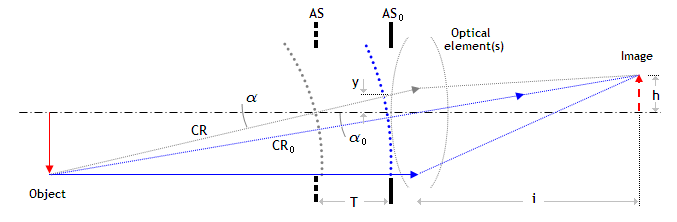FIGURE 151: The effect of aperture stop displacement on ray and wavefront geometry: AS
0 is aperture stop at the surface, and AS is axially displaced (axially centered) aperture stop; CR is the chief ray for the displaced stop, and T is stop-to-surface separation. Optical path length (OPL) of the CR itself is not an aberration factor, but the differential between the CR and other rays' OPL does result in change of wavefront aberrations.

Based on Eq. (k)-(m), lens aberration coefficients for primary spherical aberration, coma and astigmatism with the stop displaced from the surface, assuming spherical lens surface (Q=0), as a sum of the aberration coefficients for the two lens surfaces combined, are:

for primary spherical aberration, sdL = 0.125(N1J12 + N2J22δ24)

for coma, cdL = 0.5(N1J1Y1h1 + N2J2Y2h2δ23)

for astigmatism adL = -0.5(N1Y12h12 + N2Y22h22δ22)

where δ2=d2/d1 is the ratio of the effective aperture radius (i.e. height of the marginal ray of the axial cone) at the rear. vs. front surface, with the subscript L for lens (rest of parameters are unchanged). For, d2~d1, which is usually the case with sub-aperture correctors when the cone width is large relative to lens thickness, δ2 can be omitted if the resulting error is small. When calculating the P-V wavefront error, it is usually sufficiently accurate to use the d1 value for d  in Table 2, rightmost. The exact result would require calculating peak aberration coefficient for each surface, and summing them up.

Obviously, even these simplified expressions are still fairly extensive. For gaining the insight into main characteristics of lens aberrations for displaced stop, it is more practical to use expressions in terms of peak aberration coefficients which, at Gaussian focus, can be expressed as S=sd4, C=cαd3 and A=a(αd)2, for primary spherical aberration, coma and astigmatism respectively (for r=1 and t=0).

The coma peak aberration coefficient takes the form:

C = C0-4yS0         (98)

where C0 and S0 are the peak aberration coefficients for coma and spherical aberration with the stop at the lens, respectively, and

y = Tα/r        (98.1)

the relative pupil coordinate shift caused by the displaced stop, with T being the stop-to-surface separation - numerically positive when the stop is to the left from the lens - α=iα0/(i+T) the new chief ray angle, with i being the lens to image separation, and α0=h/i the chief ray angle with the stop at the lens (with h being the linear height in the image plane), and r the stop aperture radius.

Aberration coefficient of lower-order (primary) astigmatism for a single thin lens with the stop at the surface is given by:

a = -1/2f        (99)

with the P-V wavefront error at both, Gaussian and best focus, given by W=A=aα2D2/4. Doublet coefficient is a sum of its element's coefficients, and the system coefficient is a sum of the coefficient for the corrector and mirror elements. Similarly to coma, when the stop is displaced from the lens, the peak aberration coefficient, which is for the stop at the surface given by A0=a(αd)2=a(αD)2/4, becomes:

A = A0-2yC0+4yS0          (99.1)

Change in astigmatism causes change in the best image field curvature, which is now, also as the peak aberration coefficient, given by:

U = A0-yC0+2yS0         (99.2)

Relations for displaced stop - called stop shift relations - apply to optical elements in general, including mirror and lens objectives. They show that change in the stop position doesn't affect spherical aberration, but that uncorrected spherical aberration does affect both coma and astigmatism, with the latter also being affected by uncorrected coma. On the other hand, aplanatic systems (corrected for spherical aberration and coma) are unaffected by stop position. By the virtue of their function, sub-aperture correctors do have inherent (corrective) aberrations, and normally require use of stop-shift relations to calculate their off-axis aberrations.

Aberration coefficients for sub-aperture corrector can be also calculated as a sum of aberration coefficients for each surface, as outlined with field flattener lens example. It is even more time consuming and, as the above relations, only covers lower-order aberrations; that limits their usefulness for assessing aberrations of sub-aperture correctors, which commonly have significant higher-order aberration component.

A form of the sub-aperture corrector with the simplest aberration expressions is a single Schmidt plate in a converging cone, usually at some distance in front of the final focus. The aberration coefficients for lower-order spherical aberration, coma and astigmatism are s=bΔ4/8, c=-b3/2 and a=-bT2Δ2/2, respectively, where b is the aspheric coefficient, Δ is the relative plate-to-focus separation in units of the system focal length, and T is the stop (exit pupil) separation for the plate. As with the Schmidt camera, the lower-order aspheric coefficient is found from -(b/8)+ss=0, where ss is the aberration coefficient of lower-order spherical aberration for the rest of the system. The stop separation T equals the mirror-plate separation (numerically negative) in a single-mirror system with the stop at the surface. In two-mirror systems, T=E-Δƒ, where E is the exit pupil (i.e. image of the primary formed by the secondary) separation, and ƒ is the system focal length (note that both E and Δƒ are numerically positive, as is the stop-to-plate separation T for Δƒ<E).

Chromatic aberration

Chromatic aberration of a doublet corrector can be longitudinal (secondary spectrum) and lateral chromatism. As it is the case for a doublet in general, only one of the two forms can be cancelled, but the overall chromatic error can be greatly reduced by a proper choice of elements' properties (both longitudinal and lateral chromatism cancelled require each of the two elements achromatized).

For a contact, or near-contact doublet corrector, achromatizing requirements are, in first approximation, identical to those for a doublet objective. Optical power of either lens, i.e. their power of refraction, remains unchanged regardless of the object distance (i.e. steepness of the converging/diverging incident cone), as long as they satisfy the thin lens conditions. However, in a steeply diverging or, more commonly, converging cone, the height differential of refracted ray on successive lens surfaces becomes significant, giving it properties of a thick lens, even if the same lenses would act as thin lenses for near-collimated beam.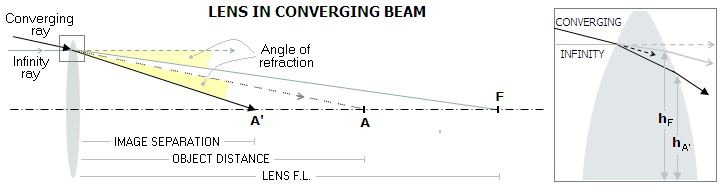FIGURE 152: According to paraxial approximation, object distance O (numerically negative for object at infinity, positive on above illustration), image separation I and focal length
ƒ of a thin lens are related as 1/ƒ=(1/I)+(1/O). Since the last two are proportional to the angle of object ray and image ray, respectively, the angle of refraction is (1/I)-(1/O)=ƒ=const. However, at large incident angles the marginal ray height at the rear vs. front surface becomes smaller (showed magnified in the inset at right), reducing its effective power relative to the front surface. Hence the chromatism contribution of such lens changes with respect to that with object at infinity, and may be compounded or partly offset by the lens, or lenses following it in the optical path. In other words, a lens system chromatically corrected for near-collimated beam, will be less than perfectly corrected in a strongly converging cone. Needless to say, monochromatic aberrations also change, in part due to the ray height differential (spherical aberration), and in part due to the stop shift (coma and astigmatism).

For sub-aperture corrector with separated elements, with the separation t defined as that between the back surfaces of the front and rear lens, the combined focal length is given by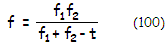with the required lens separation for achromatism given by: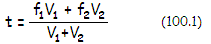where ƒ1, ƒ2 and V1, V2 are the front and rear element focal length and Abbe number, respectively. Since in this form the relation apply for object at infinity (collimated incident light at the front lens), for object at a finite distance - most common scenario with sub-aperture corrector, where the object is the image formed by the optical element preceding corrector - ƒ1 is replaced by image separation for the first lens, obtained from the Gaussian lens formula.

Achromatizing is defined as bringing two widely separate wavelengths to a common focus, thus fulfilling this condition will achromatize for selected wavelengths 1 and 2, provided that the V values used for Eq. 100.1  are those for which the refractive index is the mean value of the respective lens indici: nm=(n1+n2)/2. Consequently, the V number for each lens is V=(nm-1)/(n1-n2). The remaining longitudinal color error is reduced to the displacement of the first and second principal point of the doublet (even if consisting of a pair of thin lenses, a doublet system has properties of a thick lens), given by: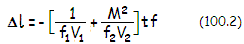with M being the wavelength-dependent transverse corrector's image magnification. Longitudinal color error l is zero for M2=-V2ƒ2/V1ƒ1. With the separation t satisfying Eq. 100.1, lateral color error reduces to:with L being the exit pupil to (final) image separation, approximated by L~-(ƒ-ic), ic being the corrector-to-image separation.

Needed lens separation for a single-glass sub-aperture doublet corrector (V1=V2=V) is, from Eq. 100.1, given by t=(ƒ1+ƒ2)/2, with its longitudinal chromatic error reduced to l=-(ƒ2+ƒ1M2)/V and the lateral error reduced to h=(ƒ1M/V)-l/L. For zero longitudinal chromatism, the lens elements' focal lengths relate as ƒ2=-ƒ1[1-(t/ƒ1)]2, due to the back focal distance becoming independent of the wavelength. Obviously, it implies that the lenses are of opposite powers, with the negative lens stronger for t>0. However, the focal length variation l is not zero, resulting in non-zero lateral chromatism.

In general, longitudinal and lateral chromatism in a separated doublet cannot be simultaneously cancelled, unless both lenses are achromatic themselves. Usually, the priority is cancelling longitudinal chromatism, while keeping lateral color low.

Using raytrace programs in designing lens correctors

As the above introduction implies, determining needed parameters of subaperture correctors is rather involved procedure. Fortunately, modern raytracing programs, such as OSLO, can make it much easier, especially with simpler corrector types. While basic knowledge of optics is still needed, following basic steps often can lead to a successful design:

(1) placing the elements in the initial position, and determining needed power to nearly cancel secondary spectrum (longitudinal chromatism);

(2) looking at the aberration coefficients for each surface, and their sum, lens surfaces are varied to minimize or cancel combined aberrations ("lens bending"), while preserving individual lens powers (i.e. longitudinal chromatic correction); in order to preserve lens power, the sum of its radii given as (1/R1)+(1/R2) for bi- and plano-lenses and (1/R1)-(1/R2) for menisci, needs to remain constant (for simplicity, radii are given as absolute values)

(3) if aberrations cannot be corrected at that particular corrector location, it should be assessed how moving it farther or closer from the focus affects the aberrations

(4) if aberrations cannot be corrected, it is likely that different lens arrangement is needed; if monochromatic aberrations are corrected but lateral color remains significant, different spacing, lens thicknesses or lens power rearrangement (if possible) should be tried, before new glass combination, with different partial dispersion, is attempted

(5) higher-order aberrations are often significant; if so, their sum of coefficient for all surfaces needs to be balanced with that of the corresponding lower-order form of opposite sign to be minimized; for spherical aberration, the two amounts are similar, absolutely, while for coma and astigmatism the lower-order form needs to be nearly twice higher (that may change if both - i.e. all four - are present); non-zero Petzval surface coefficient is compensated by about half as high lower-order astigmatism coefficient of opposite sign

9.3. Designing doublet achromat    ▐    10.1.2. Sub-aperture correctors for a single mirror